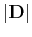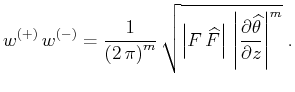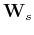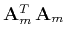Asymptotic pseudounitary stacking operatorsNext: EXAMPLES Up: Asymptotic pseudounitary stacking operators Previous: LEAST-SQUARES INVERSION AND ADJOINT

# ASYMPTOTIC PSEUDO-UNITARY OPERATOR PAIR

According to the theory of asymptotic inversion, briefly reviewed in the first part of this paper, the weighting function of the asymptotically inverse operator is inversely proportional to the weighting of the forward operator. On the other hand, the weighting in the adjoint is directly proportional to the forward weighting. This difference allows us to define a hybrid pair of operators that possess both the property of being adjoint and the property of being asymptotic inverse. It is appropriate to call a pair of operators defined in this way asymptotic pseudo-unitary. The definition of asymptotic pseudo-unitary operators follows directly from the combination of definitions (8) and (23). Splitting the derivative operatorin (8) into the product of two operators, we can write the forward operator as(24)

and its asymptotic pseudo-unitary adjoint as(25)

According to equation (10),(26)

According to equation (22),(27)

Combining equations (26) and (27) uniquely determines both weighting functions, as follows:(28)(29)

Equations (28) and (29) complete the definition of asymptotic pseudo-unitary operator pair.

The notion of pseudo-unitary operators is directly applicable in the situations where we can arbitrarily construct both forward and inverse operators. One example of such a situation is the velocity transform considered in the next section of this paper. In the more common case, the forward operator is strictly defined by the physics of a problem. In this case, we can include asymptotic inversion in the iterative least-squares inversion by means of preconditioning (Lambaré et al., 1992; Jin et al., 1992). The linear preconditioning operator should transform the forward stacking-type operator to the form (24) with the weighting function (28). Theoretically, this form of preconditioning should lead to the fastest convergence of the iterative least-squares inversion with respect to the high-frequency parts of the model.

If the forward pseudo-unitary operatorcan be related to the forward modeling operatoras, whereandare weighting operators in the data and model domains correspondingly, then preconditioning simply amounts to replacing the least-squares equation(30)

with the equation(31)

whereis the preconditioned model. The advantage of using equation (31) is in the the fact that the normal operatoris closer (asymptotically) to identity and therefore should be easier to invert than the original operatorin the least-squares solution (13).Asymptotic pseudounitary stacking operatorsNext: EXAMPLES Up: Asymptotic pseudounitary stacking operators Previous: LEAST-SQUARES INVERSION AND ADJOINT

2013-03-03